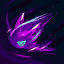League of Legends
LoL# Vex·Combos

Power SpikePQWER
Get everything you need for Vex Mid build! The highest win rate Vex runes, items, skill order and summoner spells in patch 13.1.
 Tier A- Win rate 51.1%- Pick rate 3.8%- Ban rate 6.0%- Matches 126 176-RFRWAAEQAA

Start with R then flash in then R then W then AA then E then Q then finish with AA

Featured
Hard
FeaturedEQRRW

Start with E then Q then use R twice then finish with W

AverageFWERQRAA

Flash in then W then E the R the Q then R then AA

AverageRRWEQAA

Start with R then R again then W then E then Q then finish with AA

AverageERQAARAAWAA

Start with E then R then Q then AA then R then AA then W then finish with AA

AverageAAEAAQAA

EasyAAWQEAA

Start with AA then W then Q then E then finish with AA

EasyFiltersSections